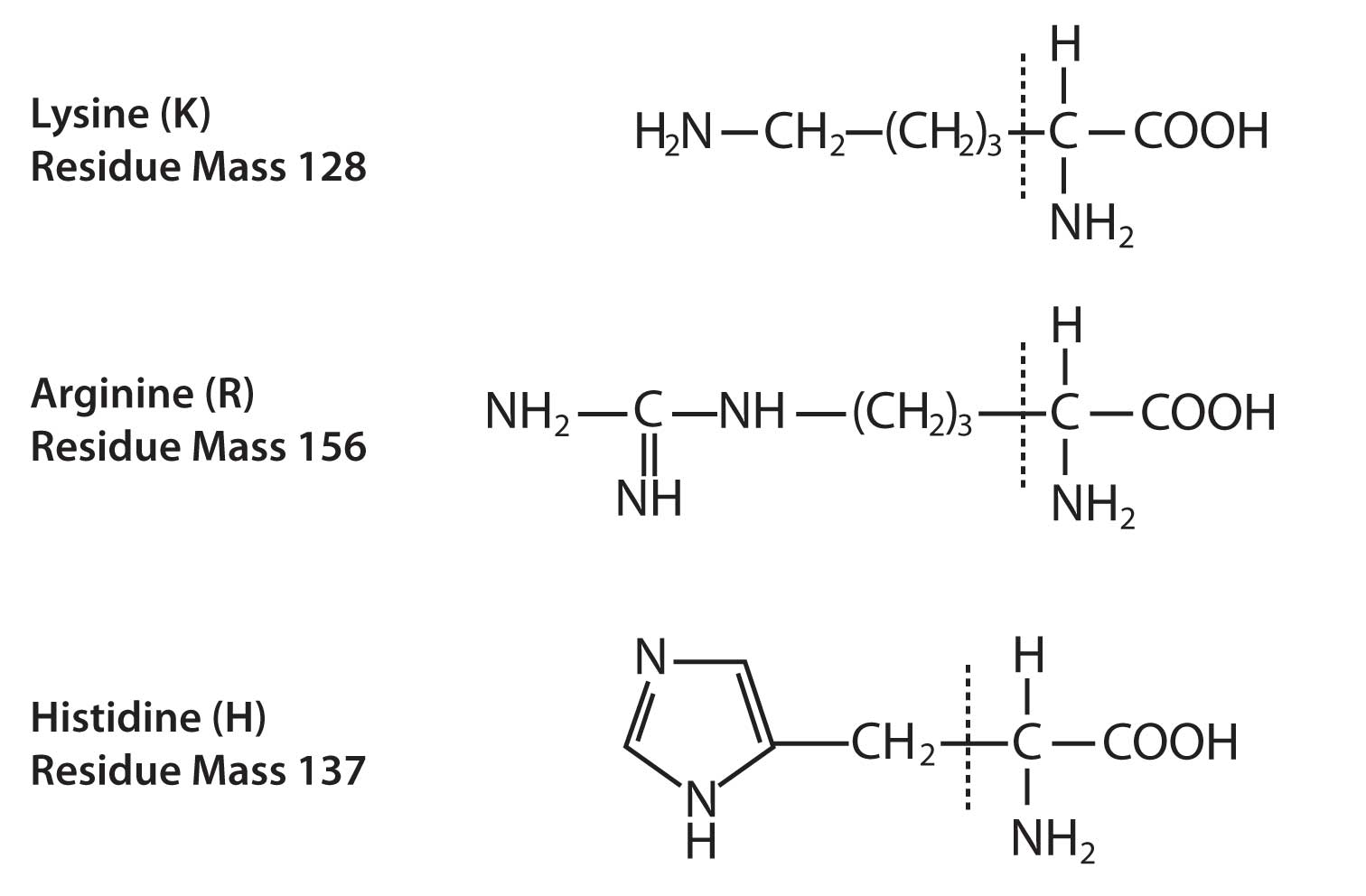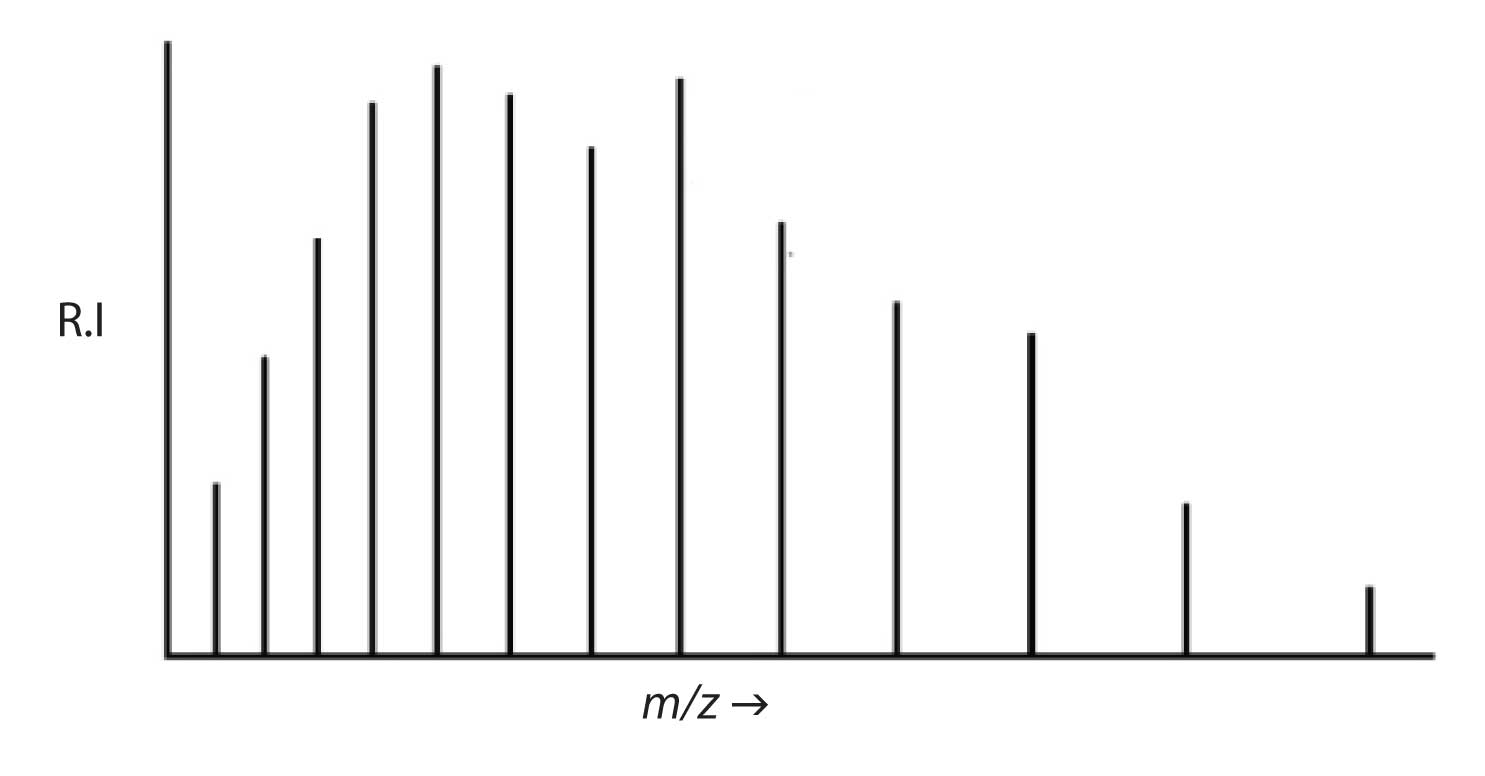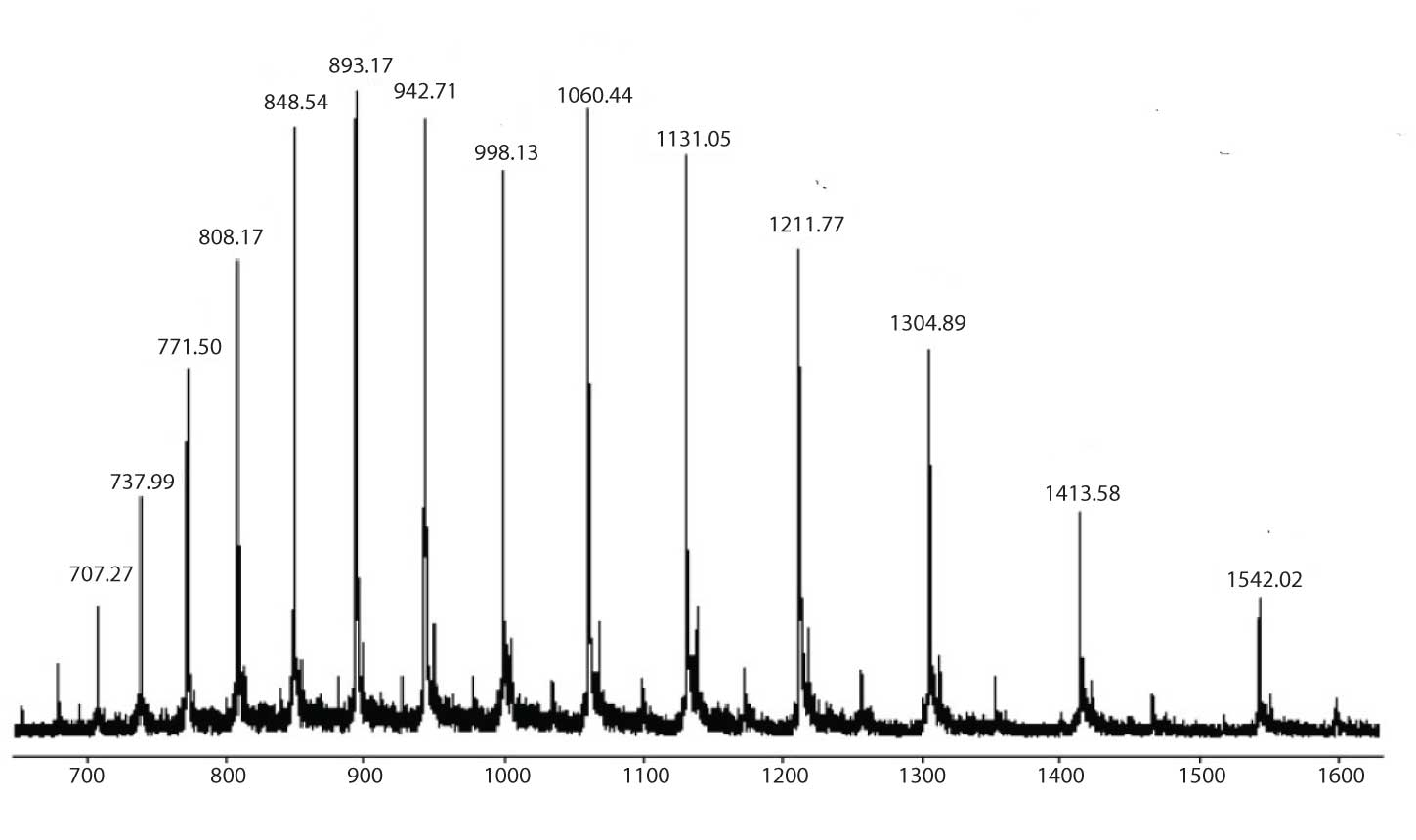# Mass Spectrometry Blog

In two previous instalments of MS Solutions, I discussed the sequencing of simple, single-charge peptide ions using MS/MS. In this and another upcoming edition I am going to discuss the use of multiple-charge ions in protein and peptide analysis.

In order to form a multiple-charge ion, an analyte molecule must have more than one site which can be ionized. In electrospray analyses, these charge locii may be any of the functional groups that we normally associate with protonation (positive charge) or deprotonation (negative charge). Amines are excellent proton acceptors and a very common site for positive charges. Carboxylic acids can donate the acidic proton from the –OH group and form a negative ion. While negative ion formation at acidic sites can occur for proteins, we most typically evaluate protein molecules in electrospray as positive ions.

A polypeptide may carry a positive charge on the amino-terminal end of the chain and on any of the basic amino acid side chains, i.e., lysine (K), arginine (R), and histidine (H). The structures for these amino acids are shown in Figure 1 with the side-chains in each case shown to the left of the vertical dashed line.Figure 1: Basic amino acids.

The ability of protein molecules to form multiple-charge ions is what allows us to use electrospray effectively even with mass analysers that have relatively limited m/z range. A protein of 100 KDa which carries 100 charges will be seen at 100 KDa/100 charges = 1000 m/z .

However, with very large numbers of charges we do not observe a single multiple-charge species in the mass spectrum (i.e., a single value for z), but rather a heterogeneity of charge. Figure 2 shows a typical multiple-charge ion spectrum of a large protein molecule. These spectra are characterized by (1) a somewhat normal distribution of intensities around the base peak and (2) increased spacing between the peaks with increasing m/z value. If these characteristics are observed it is safe to say that as we go toward higher m/z value, each peak represents one less charge than its immediately adjacent neighbour of lower m/z. It is important to note that these peaks do not represent fragment ions. Each peak represents an intact protein molecule with some number of adducted protons.Figure 2: Typical appearance of a multiple-charge ion mass spectrum of a protein.

The question we want to answer is “How many protons?” If we know the answer to this question, then we know the value for z . We can read the m/z of the peak in the spectrum and if we solve for m we can determine the molecular mass of the protein represented by this spectrum.

Starting with a molecule of mass M we can adduct z protons to create a multiple charge ion. The mass-to-charge ratio of that ion can be defined as follows, where H is the mass of a proton:

m /z = (M + z H)/z (Eq.1)

Note that m is the mass of the ion , i.e., the molecular mass of the protein plus the mass of all adducted protons. We can now solve Equation 1 for M:

m = (M + z H) (Eq. 2)

m – z H = M (Eq. 3)

M = z (m /z - H) (Eq. 4)

We can identify the m/z values of two adjacent peaks in the multiple-charge ion mass spectrum as m1/z1 and m2/z2where z1 = z2 + 1. Using Equation 1 and 4 we can derive the following:

z 2 = ((m1/z 1 )– H) / (( m2/z 2 )– (m1/z 1 )) (Eq. 5)

We can use Equation 5 to determine the number of charges on any ion in the mass spectrum by simply knowing the m/z value of that ion and the m/z value of the immediately adjacent ion of lower m/z . It is then a simple matter to determine the molecular mass M from Equation 4. If we do this for every peak in the mass spectrum and then average the results we will have a very accurate determination of the molecular mass of the analyte molecule.Figure 3: Electrospray mass spectrum of horse heart myoglobin.

In Figure 3 we see the real mass spectrum from which Figure 2 was derived. Let us choose two adjacent peaks at m/z values 1131 and 1212. We can determine the number of charges on the peak at 1212 using Equation 5:

z1212 = (1131- 1) / (1212-1131) = 14

And from Equation 4 the molecular mass is easily determined:

M = 14 (1212-1) = 16,954 Da

The sample is horse heart myoglobin and the actual molecular mass is 16,952.56 Da. When all peaks in the mass spectrum are considered and two significant figures used the calculated result is 16,951.74 Da. This represents an error of 0.82 Da or 48 ppm. The older technique of sodium dodecylsulfate detergent with polyacrylamide gel electrophoresis (SDS-PAGE) achieved molecular mass accuracy within 5-10%. It is easy to see why electrospray LC/MS has been such a valuable new tool for biotechnology.

This has been a very simple example using a very clean mass spectrum. The procedure is often called maximum entropy deconvolution. More sophisticated versions of these calculations which are applicable to “real-world” samples are available in commercial software packages targeted toward biotech users. The algorithms for these types of software packages are constantly being refined to allow faster, more accurate mass determinations, and to be able to extract multiple different protein analytes from a single mass spectrum.